# Table of Statistic Symbols with Examples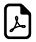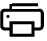## Math Statistic Symbols with Examples

Symbol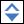Symbol NameSymbol MeaningExamples Sample Standard Deviation population samples standard deviation estimator s = 2
zx Standard Score zx = (x-x) / sx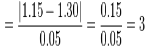X ~ Distribution of X distribution of random variable X X ~ N(0,3)
N(μ,σ2) Normal Distribution gaussian distribution X ~ N(0,3)
U(a,b) Uniform Distribution equal probability in range a,b  X ~ U(0,3)
exp(λ) Exponential Distribution f (x) = λe-λx , x≥0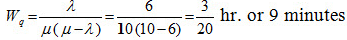gamma(c, λ) Gamma Distribution Gamma Distribution f (x) = λ c xc-1e-λx/ Γ(c), x≥0
χ 2(k) Chi-Square Distribution Chi-Square Distribution f (x) = xk/2-1e-x/2/ ( 2k/2 Γ(k/2) )
F (k1, k2) F Distribution F Distribution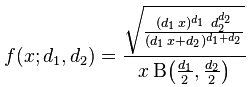Bin(n,p) Binomial Distribution Binomial Distribution f (k) = nCkpk(1-p)n-k
Poisson(λ) Poisson distribution Poisson distribution f (k) = λke-λ/ k!
Geom(p) Geometric Distribution Geometric Distribution f (k) =  p(1-p) k
HG(N,K,n) Hyper-Geometric Distribution Hyper-Geometric Distribution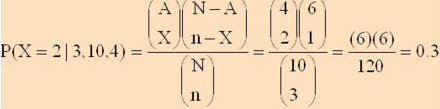Bern(p) Bernoulli Distribution Bernoulli Distribution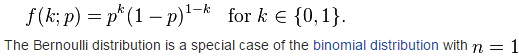P(A) Probability Function probability of event A P(A) = 0.5
P(AB) Probability of Events Intersection probability that of events A and B P(AB) = 0.5
P(A B) Probability of Events Union probability that of events A or B P(AB) = 0.5
P(A |B) Conditional Probability Function probability of event A given event B occured P(A | B) = 0.3
f (x) Probability Density Function (PDF) Probability Density Function (PDF) P(a x b)= ∫ f (x) dx
F(x) Cumulative Distribution Function (CDF) Cumulative Distribution Function (CDF) F(x) = P(X x)
μ Population Mean mean of population values μ= 10
E(X) Expectation Value expected value of random variable X E(X) = 10
E(X |Y) Conditional Expectation expected value of random variable X given Y E(X | Y=2) =5
var(X) Variance variance of random variable X var(X) = 4
σ2 Variance variance of population values σ2 = 4
std(X) Standard Deviation standard deviation of random variable X std(X) = 2
σX Standard Deviation standard deviation value of random variable X σX  = 2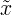Median middle value of random variable x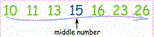cov(X,Y) Covariance covariance of random variables X and Y cov(X,Y) = 4
corr(X,Y) Correlation correlation of random variables X and Y corr(X,Y) = 0.6
ρX,Y Correlation correlation of random variables X and Y ρX,Y= 0.6
Summation summation - sum of all values in range of series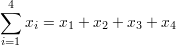∑∑ Double Summation double summation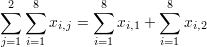Mo Mode value that occurs most frequently in population
MR Mid-Range Mid-Range MR = (xmax+xmin)/2
Md Sample Median half the population is below this value
Q1 Lower / First Quartile 25% of population are below this valueQ2 Median / Second Quartile 50% of population are below this value = median of samplesQ3 Upper / Third Guartile 75% of population are below this valuex Sample Mean average / arithmetic mean x =(2+5+9) / 3 = 5.333
s 2 Sample Variance population samples variance estimator s 2 = 4